# The oscillation

The oscillation of a mechanical oscillator can be used to measure weight. A body of unknown weight is suspended on a spring with a stiffness of 20 N times m. The resulting oscillator oscillates with a frequency of 1.6 Hz. Find the weight of the body.

m =  0.1979 kg

### Step-by-step explanation: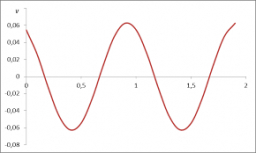Did you find an error or inaccuracy? Feel free to write us. Thank you!Tips to related online calculators
Do you want to convert time units like minutes to seconds?

## Related math problems and questions:

• Frequency of oscillation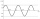A weight of 1 kg was suspended from the spring and the spring was extended by 1.5 cm. Determine the frequency of the natural oscillation of the resulting oscillator.
• Harmonic oscillator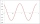Calculate the total energy of a body performing a harmonic oscillating motion if its mass is 200 g, the amplitude of the deflection is 2 cm and the frequency is 5 Hz. Round the result to 3 decimal places.
• Cuboid 5Calculate the mass of the cuboid with dimensions of 12 cm; 0.8 dm and 100 mm made from spruce wood (density = 550 kg/m3).
• Self-oscillation period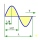The water in the vessel carried by the boy has a self-oscillation period of 0.8 s. What is the size of the boy's movement speed when the length of the boy's step is 60 cm? Give the result in m/s.
• Circular motionThe mass point regularly moves in a circle with radius r = 3.4 m angular velocity ω = 3.6 rad/s. Calculate the period, frequency, and centripetal acceleration of this movement.
• Dynamometer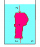What is the volume of a body that stretches a dynamometer in air, on which it is suspended by a force of 2.5 N, and if it is immersed in alcohol with a density of 800 kg/m ^ 3, does it tension the dynamometer with a force of 1.3 N?
• Free fallThe free fall body has gone 10m in the last 0.5s. Find the body speed at the moment of impact.
• Center of gravityThe mass points are distributed in space as follows - specify by coordinates and weight. Find the center of gravity of the mass points system: A1 [1; -20; 3] m1 = 46 kg A2 [-20; 2; 9] m2 = 81 kg A3 [9
• Motion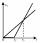The two bodies move in the same direction evenly in a straight line, at speeds of 5 cm/s and 10 cm/s. The movement of the first body started 2 seconds earlier than the movement of the second body, from a point located at a distance of 20 cm from the start
• Inclined plane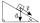1. How much work W we have to do to pull a body weighing 200 kg along an inclined plane with a length of 4 m to a total height of 1.5 m. 2. Find the force we need to exert to do this if we neglect frictional resistance. 3. Find the force we would need if
• Rotary motionFind the orbital time, rotational frequency, and angular rate of the second and minute hands on the watch.
• BomberThe aircraft flies at an altitude of 4100 m above the ground at speed 777 km/h. At what horizontal distance from the point B should be release any body from the aircraft body to fall into point B? (g = 9.81 m/s2)
• Unknown metal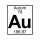The prism made of unknown metal has dimensions of 3 cm by 3 cm by 5 cm and a weight of 121 g. What metal is it? Write its chemical symbol.
• Tourist Jirka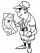Distance between the points A and B is 13.5 km. Jirka went from point A to point B unknown speed and for an unknown period of time. Back to the point A went slower by 3 km/h which means that went 20 minutes more. How long Jirka took the return journey?
• Centroid - two bodiesA body is composed of a 0.8 m long bar and a sphere with a radius of 0.1m attached so that its center lies on the longitudinal axis of the bar. Both bodies are of the same uniform material. The sphere is twice as heavy as the bar. Find the center of gravi
• Concrete columnThe concrete column of the highway bridge has the shape of a block with dimensions of 1m x 0.8m x 25m. It should be lifted by crane to a height of 20m. What is the power of his engine if the lifting takes 2 minutes?
• Brass sphereFind the weight of a brass ball with an outer radius of 12 cm, a wall thickness of 20 mm if the brass's density is 8.5 g/cm3.